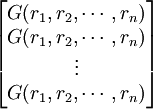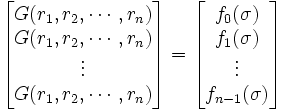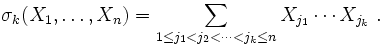## Monday, February 19, 2007

### Newton's Identities: Fundamental Theorem on Symmetric Polynomials

In today's blog, I will present the Fundamental Theorem of Symmetric Polynomials. In my previous blog, I talked about symmetric polynomials and elementary symmetric polynomials. In today's blog, I show the relationship between the two.

The content in today's blog is taken straight from Harold M. Edwards' Galois Theory.

Theorem 1: Fundamental Theorem on Symmetric Polynomials

Every symmetric polynomial in r1, ..., rn can be expressed as a polynomial in elementary symmetric polynomials σ1, ..., σn. In addition, a symmetric polynomial with integer coefficients can be expressed as a polynomial in elementary symmetric polynomials with integer coefficients.

Proof:

(1) For n=1, the symmetric polynomial is x - r1 so that we see that a1 = -r1 and σi = (-1)1(-r1)=r1

[See Definition 1, here for definition of symmetric polynomial; see Definition 2, here for definition of the kth elementary symmetric polynomial]

(2) if a1 is an integer, then r1 is an integer and σ1 is an integer.

(3) The polynomial in elementary symmetric functions is then x - σ1 which shows that the theorem is true for n=1.

(4) Let's assume that the theorem has been proved for all symmetric polynomials up to n-1.

(5) Let G(r1, r2, ..., rn) be a symmetric polynomial in n variables so that:

G(r1, r2, ..., rn) = (x - r1)*(x - r2)*...*(x - rn)

(6) Let Gi be a series of polynomials such that:

G(r1, r2, ..., rn) = G0 + G1rn + G2(rn)2 + ... + Gv(rn)v

where:

v is the highest power of rn that occurs in G and each Gi is only made up of r1, r2, ..., rn-1.

We can see that G0 is a polynomial that doesn't include rn. G1 includes all multiples of (rn)1. G2 includes all the multiples of (rn)2 and so on up until v which is defined as the highest power of rn which can be 1.

(7) Now, since G(r1, r2, ..., rn) is a symmetric polynomial, it is unchanged if any two variables ri and rj are interchanged. [See Definition 1 here for definition of symmetric polynomial]

(8) Since G(r1, r2, ..., rn) is unchanged with any interchange, so too are the polynomials defined by Gi are unchanged.

(9) This means that each Gi is itself a symmetrical polynomial on r1, r2 ... rn-1.

(10) By the inductive hypothesis in step #4, we can assume that each Gi is itself expressible as an elementary symmetric polynomial in r1, r2, ..., rn-1

(11) Let τi, τ2, ..., τn-1 denote these elementary polynomials in (n-1) variables such that [See Definition 2 here for definition of elementary symmetric polynomials]:

τ1 = r1 + r2 + ... + rn-1

τ2 = r1r2 + r1r3 + ... + rn-2rn-1
...

τn-1 = r1r2*...*rn-1

[NOTE: Each τi represents the sum of all i-combinations of 1 ... n-1. So, τ1 is the sum of all 1-combinations, τ2 is the sum of all 2-combinations, and τn-1 is the sum of all n-1 combinations (for which there is only one)].

(12) So, from the inductive hypothesis, if we let Gi(r1, ..., rn-1) represent the symmetric polynomial Gi, we can see that there exists another polynomial gi such that:

Gi(r1, ..., rn-1) = gi1, τ2, ..., τn-1)

(13) Further, from the inductive hypothesis, we know that if Gi has integer coefficients, then, so does gi.

(14) Let σ1, σ2, ..., σn be the elementary symmetric polynomials in n variables.

(15) We can now restate σi in terms of τi since:

σ1 = r1 + r2 + ... + rn = τ1 + rn

σ2 = r1r2 + r1r3 + ... + rn-1rn = τ2 + rnτ1

[Note: rn1 is equal to the sum of all (n-1) 1-combinations with rn]

σ3 = r1r2r3 + r1r2r4 + ... + rn-2rn-1rn = τ3 + rnτ2

[Note: rn1 is equal to the sum of all (n-1) 2-combinations with rn]

σ4 = τ4 + rnτ3

...

σn = r1*r2*....*rn = 0 + rn*tn-1

[Note: the main idea here is the σi represents the sum of all i-combinations of n variables. We are now equating these sums with τi which represents the sum of all i-combinations of (n-1) variables. In other words, we are now including the new combinations with rn.]

(16) We can now restate the equations in step #15 in terms of τi to get (using the basic algebraic operations of addition/subtraction to both sides of the equation):

τ1 = σ1 - rn

τ2 = σ2 - rnτ1 = σ2 - rn1 - rn) = σ2 - rnσ1 + (rn)2

τ3 = σ3 - rnτ2 = σ3 - rnσ2 + (rn)2σ1 - (rn)3.

...

τn-1 = σn-1 - rnτn-2 = σn-1 - rnσn-2 + ... + (-1)n-1(rn)n-1

(17) Finally, we can use the last equation in step #15, to get:
0 = σn - rnτn-1 = σn - rnσn-1 + ... + (-1)n(rn)n.

(18) Since we restate all the terms τi in terms of rn and σi, we can define a polynomial fi1, σ2, ..., σn-1), such that using step # 12, we have:

G(r1, r2, ..., rn) = f0 + f1n) + f2(σn)2 + ... + fμ(σn)μ

where each fi is a polynomial strictly in terms of σ1, σ2, ..., σn-1 and does not include σn.

(19) Each fi has integer coefficients if G does since:

(a) Using steps #16, we can state each fi in terms of τ1,...,τn-1

(b) From step #13, we know that τ1,...,τn-1 have integer coefficients if G has.

(20) From step #17, we get the following:

(rn)n = (rn)n-1σ1 - (rn)n-2σ2 + (rn)n-3σ3 + ... + (-1)n-1σn

(21) If μ ≥ n, then we can continuously apply the result in step #18 to the result in step #20, to get:

G(r1, r2, ..., rn) = f0(σ) + f1(σ)rn + f2(σ)(rn)2 + ... + fn-1(σ)(rn)n-1

(22) Since interchanging any values ri with rj doesn't change the value of G(r1, r2, ..., rn), then it doesn't change the value of f0(σ) + f1(σ)rn + f2(σ)(rn)2 + ... + fn-1(σ)(rn)n-1

(23) By repeatedly interchanging distinct ri and rj values where i ≠ j, we get the following n set of equations using step #21:

G(r1, r2, ..., rn) = f0 + f1r1 + f2(r1)2 + ... + fn-1(r1)n-1
G(r1, r2, ..., rn) = f0 + f1r2 + f2(r2)2 + ... + fn-1(r2)n-1
...

G(r1, r2, ..., rn) = f0 + f1rn + f2(rn)2 + ... + fn-1(rn)n-1

(24) We can think of these n equations of n terms as an n x n matrix of the form (ri)j-1 where i = the column (1 ... n) and j = the row (1 ... n).

(25) The determinant of this matrix which is a polynomial in r1, r2, ..., rn is nonzero since this is an example of the transpose of the Vandermonde Matrix [see Definition 1, here] and:

(a) From the properties of determinants, we know that det(Vn) = det(VnT) [See Theorem 9, here]

(b) The formula for the determinant of the Vandermonde matrix is (see Theorem 1, here):(c) Since by assumption r1 ≠ r2 ≠ ... ≠ rn [since each of the parameters is distinct], we can conclude that det(Vn) ≠ 0.

(26) We can think of the set of equations as a multiplication between the above matrix [(ri)j-1] and the vector [f0(σ), f1(σ), ..., fn-1(σ)] [See here for review of matrix multiplication] since:(27) But, the the set of equations is also the result of multiplying the vector[G,0,0,...,0] since:

(a) We have the following equation:(b) From step #23, we have:=(c) So we can conclude that the following two equations are equal:(28) Since the determinant in step #24 above is nonzero, the matrix is invertible (see Theorem 4, here) and we can multiply its inverse to both sides to get:(29) From this, we can conclude that:

f0 = G and f1=0, ..., fn-1=0. [See Definition 2, here]

(30) Since f0 is an elementary symmetric polynomial (see step #18 above) and since f0 has integer coefficients if G does (see step #19 above), the conclusion follows from mathematical induction (see here for review of mathematical induction).

QED

In my next blog, I will complete the proof by reviewing the formula for the determinant of the Vandermonde Matrix. In a future blog, I will show how this theorem can be used to derive Newton's identities.

References

### Newton's Identities: Symmetric Polynomials

It is very easy to build an equation that has a certain set of solutions. If we wanted to build an equation where the solutions are 1,2,3,4,5 then all we need to do is multiply out the following:

(x - 1)(x - 2)(x - 3)(x -4)(x - 5)=0

If we carry out the multiplication, we can be sure that the result is an algebraic equation of power n=5 where we have the following:

ax5 + bx4 + cx3 + dx2 + e = 0

if x = 1, 2, 3, 4, or 5. We can figure out a,b,c,d,e by carrying out the multiplication.

Let's generalize this idea. Let's assume that the roots are r1, r2, ..., rn. Further, let's call each coefficient of the final result a1, a2, ..., an.

Then we have:

xn + a1xn-1 + ... + an-1x + an = (x - r1)*(x - r2)*...*(x - rn)

If xn has a coefficient, then we can divide the entire equation by a0 to get the above result.

We call a polynomial that results in this way, a symmetric polynomial. The idea is that we can switch any ri with any rj and it doesn't change the resulting polynomial.

Definition 1: Symmetric Polynomial

Let P(x1, x2, ..., xn) be a polynomial that consists of n variables. P(x1, x2, ..., xn) is said to be a symmetric polynomial if any two of these variables can be interchanged without changing the value of the polynomial.

Examples:

The following polynomials are symmetric.

P(x1, x2) = (x1)3 + (x2)3 - 7.

P(x1, x2) = 4x1x2

P(x1, x2, x3) = x1x2x3x2 - 2x1x3 - 2x2x3

The following polynomial is not:

P(x1,x2) = x1 - x2

Now, looking at the above equation again:

xn + a1xn-1 + ... + an-1x + an = (x - r1)*(x - r2)*...*(x - rn)

We can see that:

a1 = -r1 + -r2 + ... + -rn

We know this since there are n ways to get a value of xn-1. And they are:

-r1*x*x*x*...*x -r2*x*x*x*...*x - ... -rn*x*x*x*...*x

We can also see that an = (-r1)*(-r2)*(-r3)*...*(-rn)

since this is only way to multiply the values together to get x0. We can see that there exactly C(n,0)=1 ways to include x0. There are C(n,1)=n ways to to include only x1 and C(n,2) = ways to include x2, etc.

Indeed, in each case we can see that ai is the sum of C(n,i) different terms (see Lemma 3, here for details on C(n,r) if needed):

a2 = (-r1)*(-r2) + (-r1)*(-r3) + ... + (-rn-1)*(-rn) a3 = (-r1)*(-r2)*(-r3) + ... + (-rn-2)*(-rn-1)*(-rn) ... an-1 = (-r1)*...*(-rn-1) + (-r2)*...*(-rn)

Each of these terms is itself a polynomial. They are called the elementary symmetric polynomials σi.

Definition 2: Kth Elementary Symmetric Polynomials

A kth elementary symmetric polynomial is defined as:So, we have:

σk = (-1)kak where σk is called the kth elementary symmetric polynomial in r1, ..., rn

In my next blog, I will show that for every symmetric polynomial in r1, ..., rn can be expressed as an elementary symmetric polynomial in r1, ..., rn

References